# Correlation ratio

Jump to: navigation, search

A characteristic of dependence between random variables. The correlation ratio of a random variablerelative to a random variableis the expression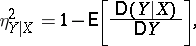whereis the variance of,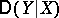is the conditional variance ofgiven, which characterizes the spread ofabout its conditional mathematical expectation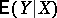for a given value of. Invariably,. The equality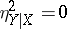corresponds to non-correlated random variables;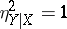if and only if there is an exact functional relationship betweenand; ifis linearly dependent on, the correlation ratio coincides with the squared correlation coefficient. The correlation ratio is non-symmetric inand, and so, together with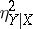, one considers(the correlation ratio ofrelative to, defined analogously). There is no simple relationship betweenand. See also Correlation (in statistics).

How to Cite This Entry:
Correlation ratio. A.V. Prokhorov (originator), Encyclopedia of Mathematics. URL: http://www.encyclopediaofmath.org/index.php?title=Correlation_ratio&oldid=13666
This text originally appeared in Encyclopedia of Mathematics - ISBN 1402006098